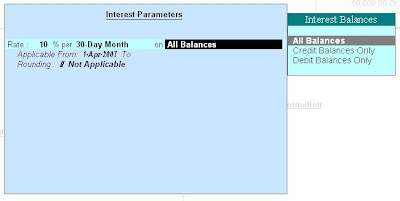Tally 9 gives you the very good facility of automatically calculation of interest on the the basis of information given by the tally user at the time of making of ledger. After this tally user can see the effect of interest collected against every ledger and every transaction. In tally 9 we can calculate both simple and compound interest. There are following steps for interest calculations in tally 9

1st step

Open tally 9 , and go to features F11 button and click it and yes in the front of activate interest calculation and advance parameters . After doing this interest calculation button will activate and accept the feature form. This is the first step which you have to pick up .

2nd step

This step divided in to two part ,
First part
At the time of creation of ledger
If you have not made the ledger then go to Account info → ledger → create → write yes in the front of activate interest calculation and set the the internal setting2nd Part

Select Ledger from ‘Accounts Info' →Ledger → Alter → select any ledger for which interest calculation has to be activated set interest calculation to yes → now provide information regarding rate of interest (%) and style as per requirements → accept the this created ledger.

Now tally 9 automatically calculate for amount receivable or amount payable

You feel tension free and just view the report

by following steps

Tally automatically calculates interest for ledgers for which interest calculations is activated. To view report,

In the gateway
select Display → Statement of Accounts → Interest Calculations → select Receivables / Payables or ledger wise.

Benefit of calculation of interest through tally 9

1. If you forget to take or give any interest , by seeing the report of interest calculation , you can remember what amount should pay.
2. This interest is corrected amount because of tally 9 database calculation.
3. This calculation of interest is very suitable for bank loan , personal loan or general business loan's interest .
4. Parameters of calculation of interest is very nice because different type of users need different type of interest calculation parameter . Some may interested to calculate interest on current credit balance or some may interest on total amount but tally 9 provide both facility in parameters of calculation of interest ..

## \$type=three\$a=hide\$cm=hide\$s=hide\$show=/2019/06/10-steps-to-become-better-investor.html\$l=hide

Name

false
ltr
item
Accounting Education: Steps for Interest Calculations in tally 9
Steps for Interest Calculations in tally 9
http://4.bp.blogspot.com/_DJEIRrK4tl4/SV3gpjA-XDI/AAAAAAAABrY/xz3m8GMIGyQ/s400/1.bmp
http://4.bp.blogspot.com/_DJEIRrK4tl4/SV3gpjA-XDI/AAAAAAAABrY/xz3m8GMIGyQ/s72-c/1.bmp
Accounting Education
http://www.svtuition.org/2009/01/steps-for-interest-calculations-in.html
http://www.svtuition.org/
http://www.svtuition.org/
http://www.svtuition.org/2009/01/steps-for-interest-calculations-in.html
true
2410664366776677676
UTF-8